# What is a Water Rocket?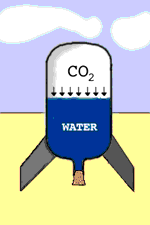A water rocket employs a compressed gas to accelerate water through its nozzle as a means of propulsion.

The water inside the motor is essentially stationary and is accelerated to the velocity at the nozzle expressed by Burnouli's equation:

Solving for Velocity: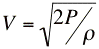(Eq. 1)

The thrust is expressed by Newton's Second Law: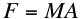Where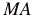is the rate of change of momentum of the water being accelerated out of the nozzle.

The Mass of the fluid flowing out of the nozzle in time dt is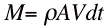Where A is the nozzle area. The Momentum (mass x velocity) is, therefore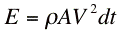If we neglect the relatively small velocity of the water inside the motor, we can say that the momentum is gained entirely within time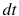So the time rate of change of the momentum (thrust) is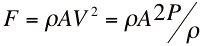by substituting for velocity from Eq. 1 above, or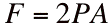updated 13-AUG-2007Question? Comment? Send A Messageupdated 23-July-2023
44.192.115.114
Copyright © 1997-2023, TRD Associates LLC